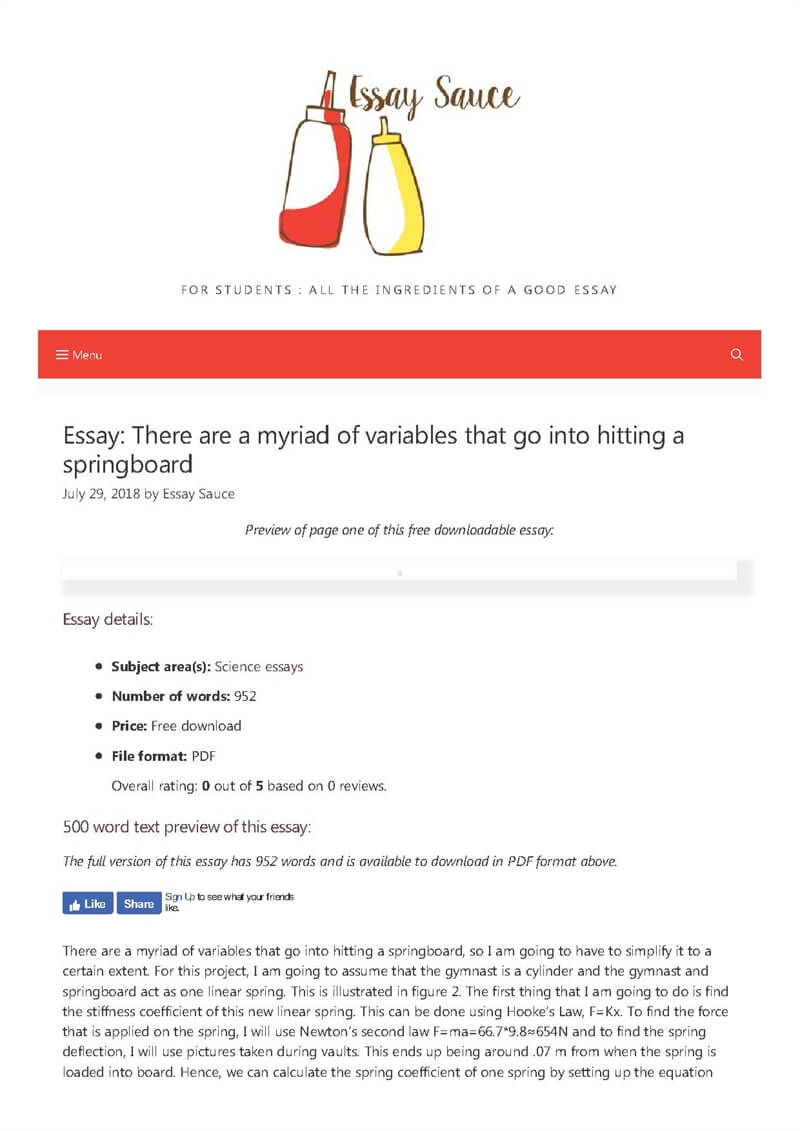# Essay: There are a myriad of variables that go into hitting a springboard### Essay details:

• Subject area(s): Science essays
• Number of words: 952
• File format: PDF
• Overall rating: 0 out of 5 based on 0 reviews.

### 500 word text preview of this essay:

The full version of this essay has 952 words and is available to download in PDF format above.

There are a myriad of variables that go into hitting a springboard, so I am going to have to simplify it to a certain extent. For this project, I am going to assume that the gymnast is a cylinder and the gymnast and springboard act as one linear spring. This is illustrated in figure 2. The first thing that I am going to do is find the stiffness coefficient of this new linear spring. This can be done using Hooke’s Law, F=Kx. To find the force that is applied on the spring, I will use Newton’s second law F=ma=66.7*9.8≈654N and to find the spring deflection, I will use pictures taken during vaults. This ends up being around .07 m from when the spring is loaded into board. Hence, we can calculate the spring coefficient of one spring by setting up the equation 654=.07K and then proceed to say K≈9343 N/m. Now this number needs to be multiplied by the number of springs to get the full coefficient. 9343*7= 65401 N/m.

Now that there is a model of what this should look like, it can be applied to vaulting. What I am trying to find is the force perpendicular or Rt as I would like to find that is propelling me forward. And as Rtas an acceleration velocity, we can use D.G. Medley’s method of finding acceleration of a rotating polar coordinate (r,Ө) where r = L+x. This equation can be expressed as at= 2drdt(dӨdt)+r(d2Өdt2). But because we know that r=L+x, and L is a constant, it can be said that drdt=dxdtand d2rdt2=d2xdt2Now we can rewrite the equation for acceleration as at=2dxdt(dӨdt)+(L+x)(d2Өdt2).

Now applying Newton’s Second Law (F=ma) we get the equation

Rt-mgcosӨ=m(2dxdt(dӨdt)+(L+x)(d2Өdt2)

But to find d2Өdt2we need to utilize an equation for torque given in “Mass-Spring Modelling of Vault Springboard Contact” (1999 page 75): T=ddt(IdӨdt). This can then be rewritten as Rt(L+x)=I(d2Өdt2)+dIdt(dӨdt) as Torque can be written as the force perpendicular/transversal times r which equals (L+x) and from the product rule of derivatives. This can then be simplified to Rt=I(d2Өdt2)+dIdt(dӨdt)L+x

Now we can use substitution to get the equation I(d2Өdt2)+dIdt(dӨdt)L+x-mgcosӨ=m(2dxdt(dӨdt)+(L+x)(d2Өdt2)

I(d2Өdt2)+dIdt(dӨdt)L+x=m(2dxdt(dӨdt)+(L+x)(d2Өdt2)+gcosӨ)

I(d2Өdt2)+dIdt(dӨdt)=m(L+x)(2dxdt(dӨdt)+(L+x)(d2Өdt2)+gcosӨ)

I(d2Өdt2)=m(L+x)(2dxdt(dӨdt)+(L+x)(d2Өdt2)+gcosӨ)-dIdt(dӨdt)

I(d2Өdt2)+m(L+x)2(d2Өdt2)=m(L+x)(2dxdt(dӨdt)+gcosӨ)-dIdt(dӨdt)

(d2Өdt2)=m(L+x)(2dxdt(dӨdt)+gcosӨ)-dIdt(dӨdt)m(L+x)2+I

Finally, I would like to know the force perpendicular when I am leaving the board to find the acceleration during the preflight. These conditions will prevail when when the velocity is changed from downward to upwards or when the Vertical component equals 0. This can be expressed by the equation:

Rv=RtsinӨ+RrcosӨ.

Then, because Rr=Kx, the equation can become

0=RtsinӨ+KxcosӨ.

With a little rearranging, this turns into

Rt=KxcosӨ-sinӨ

From the figure below, Өcan be plugged in as 80°, K as 65401 N/m, and x as 1.6-1.93=-.36 to get Rt=65401(-.36)cos80-sin80=4151.5N,

But the vertical and horizontal components are wanted so with some trigonometry, it can be found that the vertical force is 4088.4N and the horizontal force is 720.9N.

And from these forces, the vertical and horizontal accelerations can be found from F=ma and my mass being 66.68 kg. From this we get the vertical acceleration to be

This essay was submitted to us by a student in order to help you with your studies.

If you use part of this page in your own work, you need to provide a citation, as follows:

Essay Sauce, There are a myriad of variables that go into hitting a springboard. Available from:<https://www.essaysauce.com/science-essays/there-are-a-myriad-of-variables-that-go-into-hitting-a-springboard/> [Accessed 21-07-19].

### Review this essay:

Please note that the above text is only a preview of this essay. The full essay has 952 words and can be downloaded free in PDF format, using the link above.